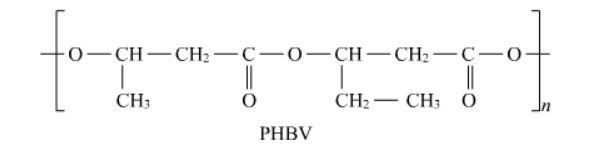Most Affordable JEE | NEET | 8,9,10 Preparation by Kota's Top IITian Doctor Faculties

# NCERT Solutions for Class 12 Chemistry Chapter 15 Polymers PDF - eSaral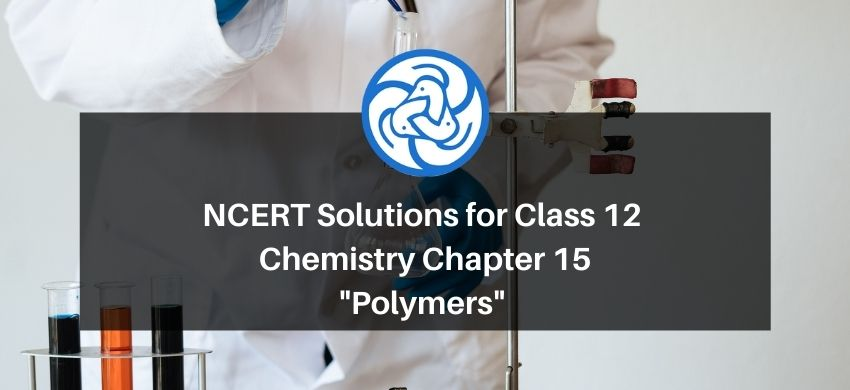# NCERT Solutions for Class 12 Chemistry Chapter 15 Polymers PDF

Hey, are you a class 12 student and looking for ways to download NCERT Solutions for Class 12 Chemistry Chapter 15 Polymers PDF? If yes. Then read this post till the end.

In this article, we have listed NCERT Solutions for Class 12 Chemistry Chapter 15 Polymers in PDF that are prepared by Kota’s top IITian Faculties by keeping Simplicity in mind.

If you want to learn and understand class 12 Chemistry Chapter 15 "Polymers" in an easy way then you can use these solutions PDF.

NCERT Solutions helps students to Practice important concepts of subjects easily. Class 12 Chemistry solutions provide detailed explanations of all the NCERT questions that students can use to clear their doubts instantly.

If you want to score high in your class 12 Chemistry Exam then it is very important for you to have a good knowledge of all the important topics, so to learn and practice those topics you can use eSaral NCERT Solutions.

So, without wasting more time Let’s start.

Question 1. What are polymers?

Solution: Polymers are high molecular mass macromolecules, which are formed by repeating structural units derived from monomers. Polymers have a high molecular mass usually ranging between $10^{3}-10^{7} \mathrm{u}$. In a polymer, various monomer units are joined by strong covalent bonds. Polymers can be natural as well as synthetic.

Some examples of polymers are Nylon 6 , Teflon and Polythene.

Question 2. How are polymers classified on the basis of structure?

Solution. On the basis of structure polymers can be classified as follows:

1. Linear polymers:

Linear polymers are formed of long straight chains. They can be depicted

as: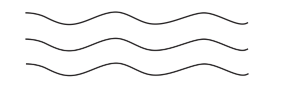For e.g., high-density polythene (HDP), polyvinyl chloride, etc.

2. Branched-chain polymers:

Branched-chain polymers are basically linear molecular chains along with some branches. These polymers are represented as:Cross-linked polymers have cross-linking strong covalent bonds between linear chains that give rise to a network-like structure. These polymers contain bi-functional and tri-functional monomers and strong covalent

bonds between various linear polymer chains. Polymers bakelite and Melmac are two examples of Cross-linked polymers.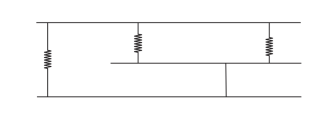Concept insight: Based on structure: Linear, Branched, cross-linked.

Question 3. Write the names of monomers of the following polymers:

Solution. (i)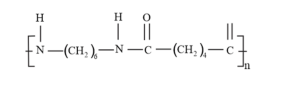(ii)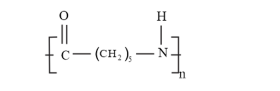(iii)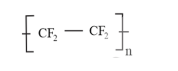Solution: (i) Consists of two monomers - Hexamethylenediamine $\left[\mathrm{H}_{2} \mathrm{~N}-\left(\mathrm{CH}_{2}\right)_{6}-\right.$ $\left.\mathrm{NH}_{2}\right]$ and adipic acid $\left[\mathrm{HOOC}-\left(\mathrm{CH}_{2}\right)_{4}-\mathrm{COOH}\right]$

(ii) The monomer is known as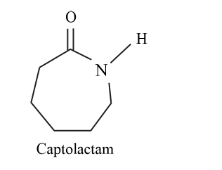(iii) Tetrafluoroethylene $\left(\mathrm{CF}_{2}=\mathrm{CF}_{2}\right)$ is the monomer.

Question 4. Classify the following as addition and condensation polymers: Terylene, Bakelite, Polyvinyl, chloride, Polythene.

Solution. Addition Polymer is formed when the molecules of the same monomer or a different monomer add together to form a polymer.

Therefore addition polymers are Polyvinyl chloride, polythene

Condensation polymers are formed by the repeated condensation of two different bi-functional or tri-functional groups leading to the elimination of simple molecules like water, hydrogen chloride.

Therefore Condensation polymers are Terylene, Bakelite.

Question 5. Explain the difference between Buna - $\mathrm{N}$ and Buna - S.

Solution.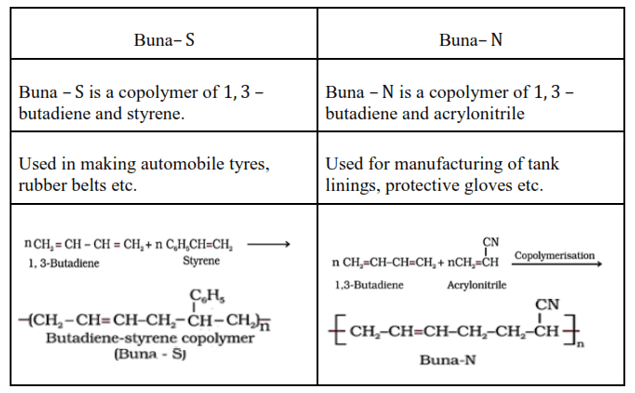Question 6. Arrange the following polymers in increasing order of their intermolecular forces

(i) $\quad$ Nylon 6, 6, Buna- S, Polythene

(ii) Nylon 6, Neoprene, Polyvinyl chloride.

Solution. On the basis of intermolecular forces of attraction, the polymers can be classified into elastomers, fibres, thermoplastic polymers and thermosetting polymers. Elastomers (rubbers) have the weakest intermolecular forces of attraction while fibers have the strongest. Plastics have intermediate intermolecular forces of attraction.

Therefore the increasing order of the intermolecular forces of the given polymers is as follows: (i) Buna $S<$ polythene $<$ Nylon 6,6

(ii) Neoprene < polyvinyl chloride < Nylon 6 Back of the chapter questions

Question 1. Explain the terms polymer and monomer.

Solution. Polymers are high molecular mass macromolecules, which are formed by repeating structural units derived from monomers. Polymers have a high molecular mass usually ranging between $10^{3}-10^{7} \mathrm{u}$. In a polymer, various monomer units are joined by strong covalent bonds. Polymers can be natural as well as synthetic.

Some examples of polymers are Polythene, rubber, Bakelite, Buna- $\mathrm{N}$

Monomers are simple, reactive molecules that combine with each other in large chains through covalent bonds to give polymers.

Some examples of monomers are ethene, propene, styrene, vinyl chloride.

Question 2. What are natural and synthetic polymers, Give two examples of each type. Solution. Polymers that are found in nature are natural polymers. These polymers are formed by plants

and animals.

Examples of natural polymers are protein, cellulose, starch, resins, etc.

Synthetic polymers are polymers made by human beings. They are derivatives of natural polymers.

Examples of synthetic polymers include plastic (polythene), synthetic fibres (nylon 6,6 ), synthetic rubbers (Buna - S).

Question 3. Distinguish between the terms homopolymer and copolymer and give an example of each.

Solution: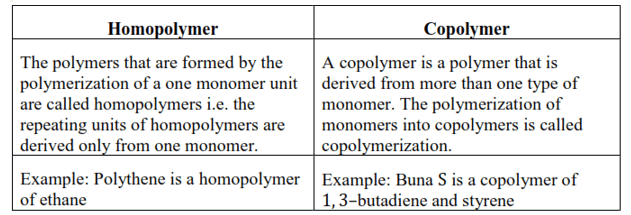Question 4. How do you explain the functionality of a monomer?

Solution: The number of binding sites that is/are present in that monomer so as to bond with other monomers to form a polymer is known as functionality of a monomer.

For example, the functionality of monomers such as ethene and propene is one and that of 1,3 -butadiene and adipic acid is two.

Question 5. Define the term polymerization.

Solution. Polymerization is the process where monomer molecules react together in a chemical reaction to form long polymer chains or three-dimensional networks. In a polymer, various monomer units are joined by strong covalent bonds.

Polymerization is broadly classified into two categories:

2. Step-Growth or Condensation Polymerization

The interaction of Hexamethylenediamine and adipic acid to form Nylon 6, 6 is an

example of a Polymerization reaction.

Question 6. Is $(\mathrm{NH}-\mathrm{CHR}-\mathrm{CO})_{\mathrm{n}}$, a homopolymer or copolymer?

Solution.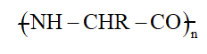is polyvinylchloride. It is a homopolymer because it is obtained from the same type of repeating monomer unit, $\mathrm{NH}_{2}-\mathrm{CHR}-\mathrm{COOH}$.

Question 7. In which classes, the polymers are classified on the basis of molecular forces?

Solution. On the basis of the magnitude of intermolecular forces of attraction that is present

in polymers, they are classified into four groups. They are:

(i) Elastomers

(ii) Fibres

(iii) Thermoplastic polymers

(iv) Thermosetting polymers

Question 8. How can you differentiate between addition and condensation polymerization?

Solution. Addition polymerization is the process of repeated addition of monomers, possessing double or triple bonds to form polymers. The monomers generally join together to form saturated polymers. For example, Addition polymerization of ethene forms polyethene.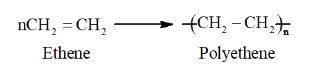The process of formation of polymers by repeated condensation reactions between two different bi-functional monomers is known as condensation polymerisation. Molecules such as water or hydrochloric acid are releases as by products in each condensation reaction.

Nylon 6, 6 is formed by the condensation polymerization of hexamethylenediamine and adipic acid.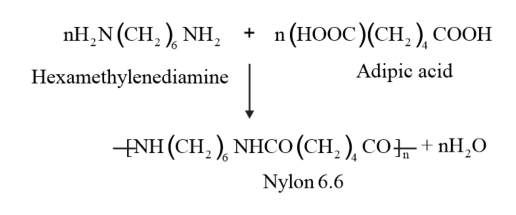Question 9. Explain the term copolymerization and given two examples.

Solution. A copolymer is a polymer that is derived from more than one type of monomer. The polymerization of monomers into copolymers is called copolymerization. Multiple units of each monomer are present in the copolymer.

Example: Buna S is a copolymer of 1, 3–butadiene and styrene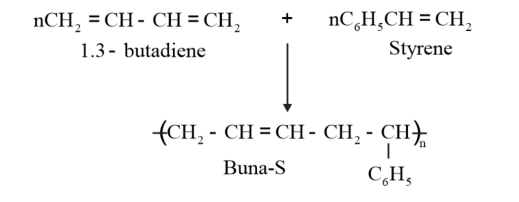Nylon 6,6 is a copolymer formed by copolymerisation of hexamethylene diamine and adipic acid.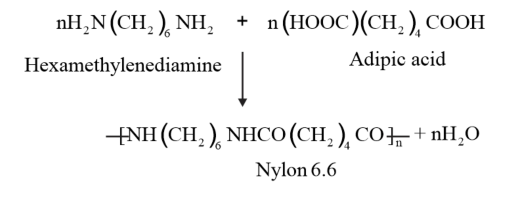Question 10. Write the free radical mechanism for the polymerization of ethene.

Solution. Polymerization of ethene to polythene includes heating or exposing to light a mixture of ethene with a small amount of benzoyal peroxide as the initiator.

The reactions involved in this process are:

Chain initiation step: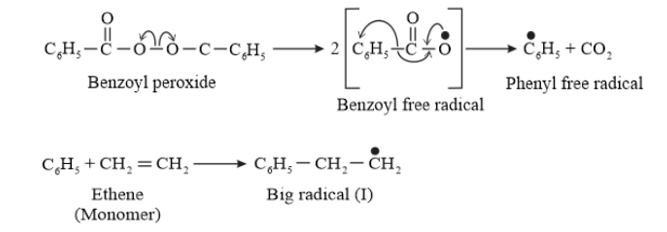Chain propagating step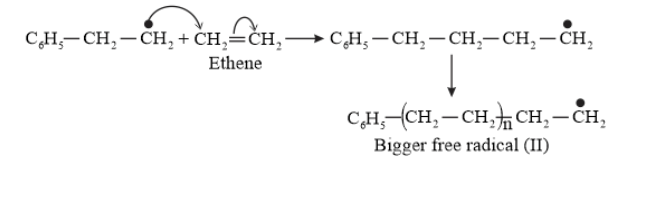Chain Terminating Step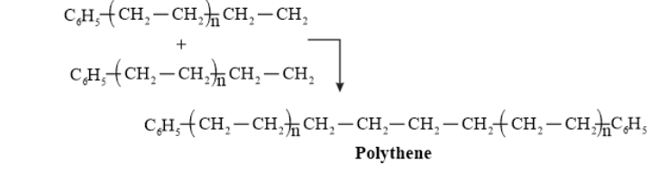Question 11. Define thermoplastics and thermosetting polymers with two examples of each.

Solution. Thermoplastic polymers are linear (slightly branched) long-chain polymers, which can be repeatedly softened on heating and hardened on cooling. Hence, they can be modified again and again. They possess intermolecular forces of attraction whose magnitude is intermediate between elastomers and fibers. Examples of thermoplastics are polythene, polystyrene, polyvinyl etc.

Thermosetting polymers are cross-linked and heavily branched polymers which on heating undergo extensive cross-linking in moulds and become infusible. These polymers cannot be softened again on heating. Examples of thermosetting plastics are bakelite, urea-formaldehyde resins.

Question 12. Write the monomers used for getting the following polymers.

(i) Polyvinyl chloride

(ii) Teflon

(iii) Bakelite

Solution. Monomers used for getting the polymers are:

(i) $\quad$ Vinyl chloride $\left(\mathrm{CH}_{2}=\mathrm{CHCI}\right)$

(ii) $\quad$ Tetrafluoroethylene $\left(\mathrm{CF}_{2}=\mathrm{CF}_{2}\right)$

(iii) Formaldehyde (HCHO) and phenol $\left(\mathrm{C}_{6} \mathrm{H}_{5} \mathrm{OH}\right)$

Question 13. Write the name and structure of one of the common initiators used in free radical addition polymerisation.

Solution. Benzoyl peroxide is one of the most common initiator used in free radical addition polymerization. The structure of Benzoyl peroxide is: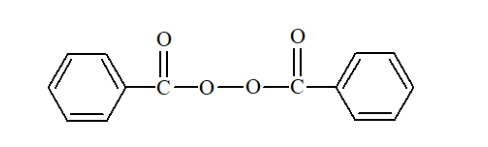Question 14. How does the presence of double bonds in rubber molecules influence their structure and reactivity?

Solution. Natural rubber is a linear cis-polyisoprene in which the double bonds are present between $C_{2}$ and $C_{3}$ of the isoprene units.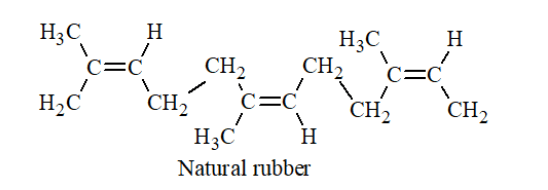Because of this configuration, It increases the elasticity of the rubber, as the chains are held together by weak van der Waals forces and have coiled structure.

Question 15. Discuss the main purpose of vulcanization of rubber.

Solution. Vulcanization of natural rubber is done to improve properties such as hardness, toughness and tensile strength. In Vulcanization, a mixture of raw rubber with sulphur and appropriate additives is heated at a temperature range between $373 \mathrm{~K}$ and $415 \mathrm{~K}$. Some Additives like Zinc oxide are used to accelerate the process since this is a slow process. During this process, sulphur cross-links are formed which makes incereases rubber's hardness and toughness with greater tensile strength. This vulcanized rubber has excellent elasticity, property of low water absorption and resistance to oxidation \& organic solvents.

Question 16. What are the monomeric repeating units of Nylon - 6 and Nylon $-6,6 ?$

Solution. The monomeric repeating unit of nylon 6 is $\left[\mathrm{NH}-\left(\mathrm{CH}_{2}\right)_{5}-\mathrm{CO}\right]$, which is derived from Caprolactam.

The monomeric repeating unit of nylon 6,6 is $\left[\mathrm{NH}-\left(\mathrm{CH}_{2}\right)_{6}-\mathrm{NH}-\mathrm{CO}-\right.$ $\left.\left(\mathrm{CH}_{2}\right)_{4}-\mathrm{CO}\right]$, which is derived from hexamethylenediamine and adipoyl chloride.

Question 17. Write the names and structures of the monomers of the following polymers:

Solution. (i) Buna-S

(ii) Buna-N

(iii) Dacron

(iv) Neoprene

Solution: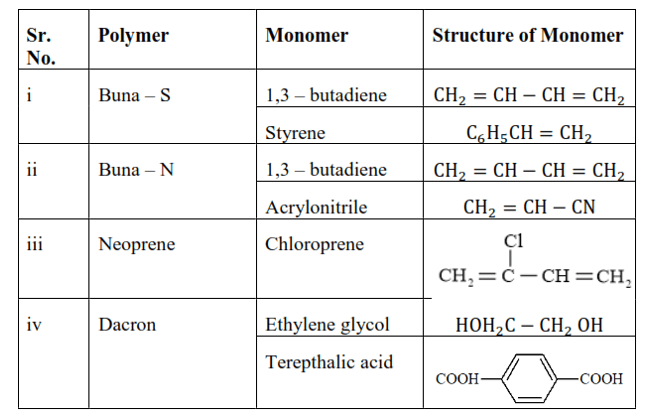Question 18. Identify the monomer in the following polymeric structures.

(i)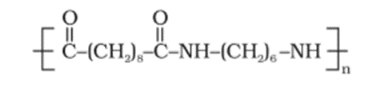(ii)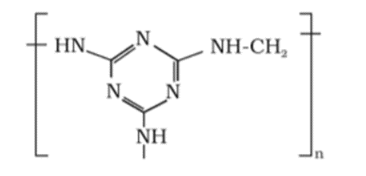Solution:

(i) The moners of the given polymeric structure are decanoic acid $\left[\mathrm{HOOC}-\left(\mathrm{CH}_{2}\right)_{8}-\mathrm{COOH}\right]$ and hexamethylene diamine $\left[\mathrm{H}_{2} \mathrm{~N}-\left(\mathrm{CH}_{2}\right)_{6}-\right.$ $\left.\mathrm{NH}_{2}\right]$.

(ii) The monomers of the given polymeric structure are melamine and formaldehyde.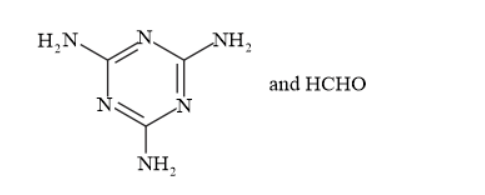Question 19. How is dacron obtained from ethylene glycol and terephthalic acid?

Solution. The condensation polymerization of ethylene glycol and terephthalic acid produces dacron. The temperature maintained for the reaction is 420 to $460 \mathrm{~K}$ and the catalyst used is zinc acetate-antimony trioxide.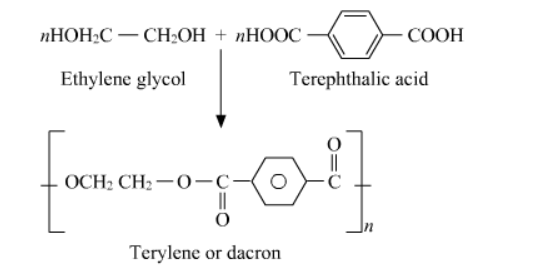Question 20. What is a biodegradable polymer? Give an example of a biodegradable aliphatic polyester

Solution. A polymer that can be decomposed by bacteria is called a biodegradable polymer. Biodegradable polymers get degraded into simpler components like water, carbon dioxide, Nitrogen etc. upon decomposition by the action of living organisms.

Poly $-\beta$-hydroxybutyrate $-$ co $-\beta$-hydroxyvalerate (PHBV) is an example of biodegradable aliphatic polyester.